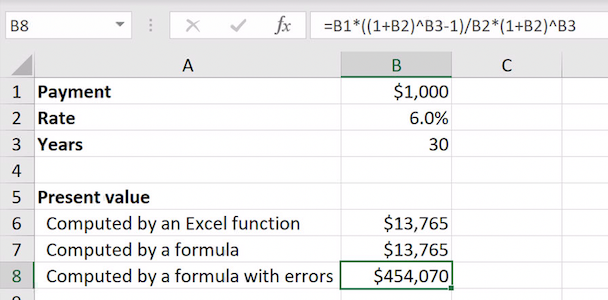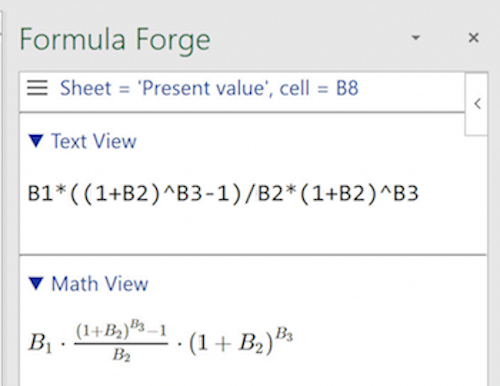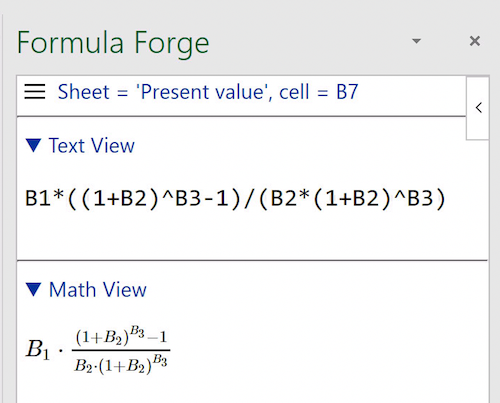# Uses Cases

## Use Case: Dealing with Parentheses

Here is an Excel spreadsheet with three computations for the present value of an annuity, one using Excel's `PV` function and two using formulas. An error with parentheses in the formula for cell `B8` produces a value that is clearly wrong, but the error is tough to see.An incorrectly parenthesized formula

Let's use Formula Forge to find the error. We select cell `B8` and click the `Get Formula` button on Excel's `Formulas` ribbon to see a pane with three views of the offending formula, the first two of which are shown here.The `Formula Forge` pane

The math view, which typesets the formula, clearly shows the error: the third term in the product belongs in the denominator of the fraction. We can correct this error by inserting parentheses around everything following the division sign in the formula. Cell `B7` already contains the correct formula, as shown here.The correctly parenthesized formula